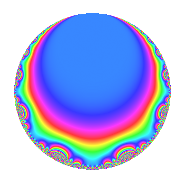# Properties

 Label 4012.2.a.dLevel 4012 Weight 2 Character orbit 4012.a Self dual Yes Analytic conductor 32.036 Analytic rank 1 Dimension 2 CM No Inner twists 1

# Related objects

## Newspace parameters

 Level: $$N$$ = $$4012 = 2^{2} \cdot 17 \cdot 59$$ Weight: $$k$$ = $$2$$ Character orbit: $$[\chi]$$ = 4012.a (trivial)

## Newform invariants

 Self dual: Yes Analytic conductor: $$32.0359812909$$ Analytic rank: $$1$$ Dimension: $$2$$ Coefficient field: $$\Q(\sqrt{5})$$ Coefficient ring: $$\Z[a_1, \ldots, a_{5}]$$ Coefficient ring index: $$1$$ Fricke sign: $$1$$ Sato-Tate group: $\mathrm{SU}(2)$

## $q$-expansion

Coefficients of the $$q$$-expansion are expressed in terms of $$\beta = \frac{1}{2}(1 + \sqrt{5})$$. We also show the integral $$q$$-expansion of the trace form.

 $$f(q)$$ $$=$$ $$q$$ $$-2 \beta q^{3}$$ $$+ ( 2 - \beta ) q^{5}$$ $$-3 q^{7}$$ $$+ ( 1 + 4 \beta ) q^{9}$$ $$+O(q^{10})$$ $$q$$ $$-2 \beta q^{3}$$ $$+ ( 2 - \beta ) q^{5}$$ $$-3 q^{7}$$ $$+ ( 1 + 4 \beta ) q^{9}$$ $$+ \beta q^{11}$$ $$+ ( -2 - \beta ) q^{13}$$ $$+ ( 2 - 2 \beta ) q^{15}$$ $$- q^{17}$$ $$+ ( -1 + 2 \beta ) q^{19}$$ $$+ 6 \beta q^{21}$$ $$+ ( 4 - \beta ) q^{23}$$ $$-3 \beta q^{25}$$ $$+ ( -8 - 4 \beta ) q^{27}$$ $$+ ( 1 + 3 \beta ) q^{29}$$ $$-9 q^{31}$$ $$+ ( -2 - 2 \beta ) q^{33}$$ $$+ ( -6 + 3 \beta ) q^{35}$$ $$+ 3 q^{37}$$ $$+ ( 2 + 6 \beta ) q^{39}$$ $$+ 9 q^{41}$$ $$+ ( -3 + \beta ) q^{43}$$ $$+ ( -2 + 3 \beta ) q^{45}$$ $$+ ( -1 + 3 \beta ) q^{47}$$ $$+ 2 q^{49}$$ $$+ 2 \beta q^{51}$$ $$+ ( -5 + 6 \beta ) q^{53}$$ $$+ ( -1 + \beta ) q^{55}$$ $$+ ( -4 - 2 \beta ) q^{57}$$ $$+ q^{59}$$ $$+ ( 1 + 4 \beta ) q^{61}$$ $$+ ( -3 - 12 \beta ) q^{63}$$ $$+ ( -3 + \beta ) q^{65}$$ $$+ ( -1 - 6 \beta ) q^{67}$$ $$+ ( 2 - 6 \beta ) q^{69}$$ $$+ ( 5 + 5 \beta ) q^{71}$$ $$+ ( -7 + 10 \beta ) q^{73}$$ $$+ ( 6 + 6 \beta ) q^{75}$$ $$-3 \beta q^{77}$$ $$+ ( -8 + 5 \beta ) q^{79}$$ $$+ ( 5 + 12 \beta ) q^{81}$$ $$+ ( 1 - 4 \beta ) q^{83}$$ $$+ ( -2 + \beta ) q^{85}$$ $$+ ( -6 - 8 \beta ) q^{87}$$ $$+ ( 1 + 7 \beta ) q^{89}$$ $$+ ( 6 + 3 \beta ) q^{91}$$ $$+ 18 \beta q^{93}$$ $$+ ( -4 + 3 \beta ) q^{95}$$ $$+ ( -13 + 5 \beta ) q^{97}$$ $$+ ( 4 + 5 \beta ) q^{99}$$ $$+O(q^{100})$$ $$\operatorname{Tr}(f)(q)$$ $$=$$ $$2q$$ $$\mathstrut -\mathstrut 2q^{3}$$ $$\mathstrut +\mathstrut 3q^{5}$$ $$\mathstrut -\mathstrut 6q^{7}$$ $$\mathstrut +\mathstrut 6q^{9}$$ $$\mathstrut +\mathstrut O(q^{10})$$ $$2q$$ $$\mathstrut -\mathstrut 2q^{3}$$ $$\mathstrut +\mathstrut 3q^{5}$$ $$\mathstrut -\mathstrut 6q^{7}$$ $$\mathstrut +\mathstrut 6q^{9}$$ $$\mathstrut +\mathstrut q^{11}$$ $$\mathstrut -\mathstrut 5q^{13}$$ $$\mathstrut +\mathstrut 2q^{15}$$ $$\mathstrut -\mathstrut 2q^{17}$$ $$\mathstrut +\mathstrut 6q^{21}$$ $$\mathstrut +\mathstrut 7q^{23}$$ $$\mathstrut -\mathstrut 3q^{25}$$ $$\mathstrut -\mathstrut 20q^{27}$$ $$\mathstrut +\mathstrut 5q^{29}$$ $$\mathstrut -\mathstrut 18q^{31}$$ $$\mathstrut -\mathstrut 6q^{33}$$ $$\mathstrut -\mathstrut 9q^{35}$$ $$\mathstrut +\mathstrut 6q^{37}$$ $$\mathstrut +\mathstrut 10q^{39}$$ $$\mathstrut +\mathstrut 18q^{41}$$ $$\mathstrut -\mathstrut 5q^{43}$$ $$\mathstrut -\mathstrut q^{45}$$ $$\mathstrut +\mathstrut q^{47}$$ $$\mathstrut +\mathstrut 4q^{49}$$ $$\mathstrut +\mathstrut 2q^{51}$$ $$\mathstrut -\mathstrut 4q^{53}$$ $$\mathstrut -\mathstrut q^{55}$$ $$\mathstrut -\mathstrut 10q^{57}$$ $$\mathstrut +\mathstrut 2q^{59}$$ $$\mathstrut +\mathstrut 6q^{61}$$ $$\mathstrut -\mathstrut 18q^{63}$$ $$\mathstrut -\mathstrut 5q^{65}$$ $$\mathstrut -\mathstrut 8q^{67}$$ $$\mathstrut -\mathstrut 2q^{69}$$ $$\mathstrut +\mathstrut 15q^{71}$$ $$\mathstrut -\mathstrut 4q^{73}$$ $$\mathstrut +\mathstrut 18q^{75}$$ $$\mathstrut -\mathstrut 3q^{77}$$ $$\mathstrut -\mathstrut 11q^{79}$$ $$\mathstrut +\mathstrut 22q^{81}$$ $$\mathstrut -\mathstrut 2q^{83}$$ $$\mathstrut -\mathstrut 3q^{85}$$ $$\mathstrut -\mathstrut 20q^{87}$$ $$\mathstrut +\mathstrut 9q^{89}$$ $$\mathstrut +\mathstrut 15q^{91}$$ $$\mathstrut +\mathstrut 18q^{93}$$ $$\mathstrut -\mathstrut 5q^{95}$$ $$\mathstrut -\mathstrut 21q^{97}$$ $$\mathstrut +\mathstrut 13q^{99}$$ $$\mathstrut +\mathstrut O(q^{100})$$

## Embeddings

For each embedding $$\iota_m$$ of the coefficient field, the values $$\iota_m(a_n)$$ are shown below.

For more information on an embedded modular form you can click on its label.

Label $$\iota_m(\nu)$$ $$a_{2}$$ $$a_{3}$$ $$a_{4}$$ $$a_{5}$$ $$a_{6}$$ $$a_{7}$$ $$a_{8}$$ $$a_{9}$$ $$a_{10}$$
1.1
 1.61803 −0.618034
0 −3.23607 0 0.381966 0 −3.00000 0 7.47214 0
1.2 0 1.23607 0 2.61803 0 −3.00000 0 −1.47214 0
 $$n$$: e.g. 2-40 or 990-1000 Significant digits: Format: Complex embeddings Normalized embeddings Satake parameters Satake angles

## Inner twists

This newform does not admit any (nontrivial) inner twists.

## Atkin-Lehner signs

$$p$$ Sign
$$2$$ $$-1$$
$$17$$ $$1$$
$$59$$ $$-1$$

## Hecke kernels

This newform can be constructed as the intersection of the kernels of the following linear operators acting on $$S_{2}^{\mathrm{new}}(\Gamma_0(4012))$$:

 $$T_{3}^{2}$$ $$\mathstrut +\mathstrut 2 T_{3}$$ $$\mathstrut -\mathstrut 4$$ $$T_{5}^{2}$$ $$\mathstrut -\mathstrut 3 T_{5}$$ $$\mathstrut +\mathstrut 1$$